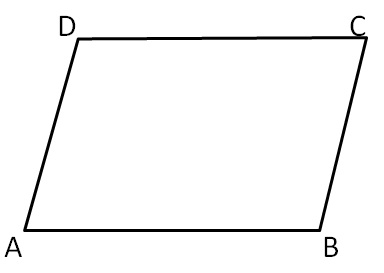In parallelogram

• Opposite angles are equal

∠A = ∠C and ∠B = ∠D

∠A + ∠B = 180°

∠A + ∠D = 180°

∠C + ∠D = 180°

∠B + ∠C = 180°

1. Chapter 3 Class 8 Understanding Quadrilaterals
2. Concept wise
3. Angles of Parallelogram

Angles of Parallelogram